• 卷积原理 池化-特征降维 最大池化层 平均池化层 1X1卷积 池化是对每个feature map的卷积，没有对通道的交互，1X1卷积对通道进行线性组合 对于单通道的feature map和单个卷积核之间的卷积来说，题主的理解...
卷积原理

池化-特征降维
最大池化层平均池化层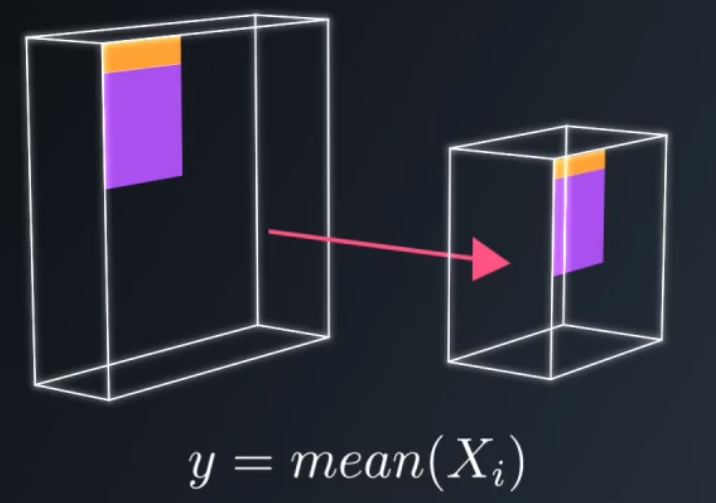1X1卷积

池化是对每个feature map的卷积，没有对通道的交互，1X1卷积对通道进行线性组合

对于单通道的feature map和单个卷积核之间的卷积来说，题主的理解是对的，CNN里的卷积大都是多通道的feature map和多通道的卷积核之间的操作（输入的多通道的feature map和一组卷积核做卷积求和得到一个输出的feature map），如果使用1x1的卷积核，这个操作实现的就是多个feature map的线性组合，可以实现feature map在通道个数上的变化。接在普通的卷积层的后面，配合激活函数，就可以实现network in network的结构

升降维度

Inception模块

增加网络深度和宽度的同时减少参数。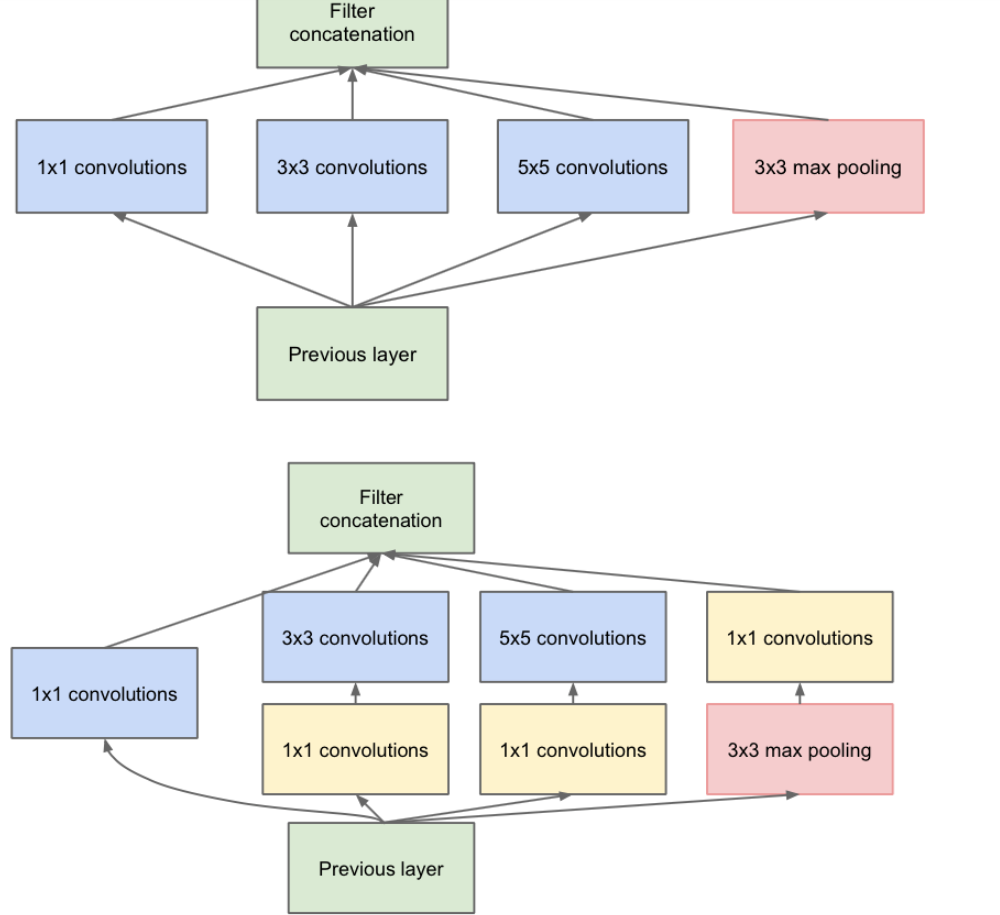Conv2D(filters, kernel_size, strides, padding, activation='relu', input_shape)
参数
必须传递以下参数：

filters

-----------------
filters 过滤器个数
kernel_size (方)卷积窗口的高宽(数字或元组)
strides  卷积步长 (数字或元组)

1×1 卷积核的作用？（附实例）：https://zhuanlan.zhihu.com/p/35814486
扩展知乎话题：卷积神经网络中用1*1 卷积有什么作用或者好处呢？：https://www.zhihu.com/question/56024942
吴恩达DeepLearning.ai视频教程 《
Networks in Networks and 1x1 Convolutions》
1*1的卷积核与Inception：https://blog.csdn.net/a1154761720/article/details/53411365


展开全文• caffe卷积原理
原理说明

caffe中的卷积计算是将卷积核矩阵和输入图像矩阵变换成两个大的矩阵，然后进行相乘得到的结果，如下图所示。具体参数如下：

Feature Matrix

Feature Matrix的大小为K×N<!--//--><![CDATA[//><!--
K×N
//--><!]]>，各项参数如下：

//--><!]]>
imageh<!--//--><![CDATA[//><!--
image_h
//--><!]]>：输入图像高度，即N×C×W×H<!--//--><![CDATA[//><!--
N\times C\times W\times H
//--><!]]>中的H<!--//--><![CDATA[//><!--
H
//--><!]]>
imagew<!--//--><![CDATA[//><!--
image_w
//--><!]]>：输入图像宽度，即N×C×W×H<!--//--><![CDATA[//><!--
N\times C\times W\times H
//--><!]]>中的W<!--//--><![CDATA[//><!--
W
//--><!]]>
//--><!]]>个单位长度
//--><!]]>个单位长度
kernelh<!--//--><![CDATA[//><!--
kernel_h
//--><!]]>：卷积核的高度
kernelw<!--//--><![CDATA[//><!--
kernel_w
//--><!]]>：卷积核的宽度
strideh<!--//--><![CDATA[//><!--
stride_h
//--><!]]>：高度方向的滑动步长
stridew<!--//--><![CDATA[//><!--
stride_w
//--><!]]>：宽度方向的滑动步长

因此，N<!--//--><![CDATA[//><!--
N
//--><!]]>为输出图像大小的长宽乘积，也是卷积核在输入图像上滑动可截取的最大特征数。

Filter Matrix

Filter Matrix的大小为M×K<!--//--><![CDATA[//><!--
M\times K
//--><!]]>，其中M<!--//--><![CDATA[//><!--
M
//--><!]]>是卷积核的个数，K=k×k<!--//--><![CDATA[//><!--
K=k\times k
//--><!]]>，等于卷积核的大小，即Filter Matrix矩阵每行为一个卷积核向量（是将二维的卷积核转化成一维），总共有M<!--//--><![CDATA[//><!--
M
//--><!]]>行，表示有M<!--//--><![CDATA[//><!--
M
//--><!]]>个卷积核。

Output Matrix

Output Matrix是Filter Matrix和Feature Matrix的乘积，是一个M×N<!--//--><![CDATA[//><!--
M\times N
//--><!]]>的矩阵，其中每一行代表一个输出图像即feature map，总共有M<!--//--><![CDATA[//><!--
M
//--><!]]>个输出图像（输出图像数目等于卷积核数目）

caffe卷积原理图参考文献

caffe的卷积原理 知乎上贾扬清大神的回答
展开全文caffe

千次阅读 2019-08-22 18:37:31
原文链接：Winograd卷积原理 | Hey~YaHei!
比如，
直接实现一个 $m$ 输出、$r$ 参数的FIR滤波器 $F(m,r)$，一共需要 $m \times r$ 次乘法运算；
但使用Winograd算法，忽略变换过程的话，仅仅需要 $m + r - 1$ 次乘法运算。
$F(2,3)$
如果直接计算 $F(2,3)$：
$F(2,3)=\left[\begin{array}{lll}{d_{0}} & {d_{1}} & {d_{2}} \\ {d_{1}} & {d_{2}} & {d_{3}}\end{array}\right]\left[\begin{array}{l}{g_{0}} \\ {g_{1}} \\ {g_{2}}\end{array}\right]=\left[\begin{array}{l}{d_0g_0+d_1g_1+d_2g_2} \\ {d_1g_0+d_2g_1+d_3g_2}\end{array}\right]$
其中，
$d_0, d_1, d_2$和$d_1, d_2, d_3$为连续的两个输入序列；
$g_0, g_1, g_2$为FIR的三个参数；
这个过程一共需要6次乘法，和4次加法
而Winograd算法指出，$F(2,3)$ 可以这样计算：
$F(2,3)=\left[\begin{array}{lll}{d_{0}} & {d_{1}} & {d_{2}} \\ {d_{1}} & {d_{2}} & {d_{3}}\end{array}\right]\left[\begin{array}{l}{g_{0}} \\ {g_{1}} \\ {g_{2}}\end{array}\right]=\left[\begin{array}{l}{m_{1}+m_{2}+m_{3}} \\ {m_{2}-m_{3}-m_{4}}\end{array}\right]$
其中，
$\begin{array}{ll}{m_{1}=\left(d_{0}-d_{2}\right) g_{0}} & {m_{2}=\left(d_{1}+d_{2}\right) \frac{g_{0}+g_{1}+g_{2}}{2}} \\ {m_{4}=\left(d_{1}-d_{3}\right) g_{2}} & {m_{3}=\left(d_{2}-d_{1}\right) \frac{g_{0}-g_{1}+g_{2}}{2}}\end{array}$
该用矩阵运算可以表示成：
$Y=A^{T}\left[(G g) \odot\left(B^{T} d\right)\right]$
其中，$\odot$表示点乘，而
$B^{T}=\left[\begin{array}{rrrr}{1} & {0} & {-1} & {0} \\ {0} & {1} & {1} & {0} \\ {0} & {-1} & {1} & {0} \\ {0} & {1} & {0} & {-1}\end{array}\right], G=\left[\begin{array}{rrr}{1} & {0} & {0} \\ {\frac{1}{2}} & {\frac{1}{2}} & {\frac{1}{2}} \\ {\frac{1}{2}} & {-\frac{1}{2}} & {\frac{1}{2}} \\ {0} & {0} & {1}\end{array}\right], A^{T}=\left[\begin{array}{rrrr}{1} & {1} & {1} & {0} \\ {0} & {1} & {-1} & {-1}\end{array}\right]$
$g=\left[\begin{array}{lll}{g_{0}} & {g_{1}} & {g_{2}}\end{array}\right]^{T}, d=\left[\begin{array}{llll}{d_{0}} & {d_{1}} & {d_{2}} & {d_{3}}\end{array}\right]^{T}$
这……似乎反而把问题变得十分复杂，但实际上它的计算量却真真切切地减少了——

由于$g$是固定的FIR滤波器参数，那么$Gg$可以提前计算并得到一个 $4\times1$ 的列向量
由于$d$是变化的输入序列，所以每次计算FIR的时候都需要对输入$d$做一个变换$B^Td$，得到一个 $4 \times 1$ 的列向量，这个过程需要4次加法（注意$B^T$矩阵的元素值）
然后$Gg$和$B^Td$进行点乘，共计4次乘法
最后$A^T$与$[(Gg)\odot(B^Td)]$做乘法，共计4次加法

过程1可以提前完成，变换过程2和计算过程3、4共计4次乘法和8次加法，相比于直接FIR的6次乘法、4次加法，乘法次数下降为原来的$\frac{2}{3}$（推广到一般情况，直接FIR跟Winograd的乘法次数分别是$m \times r$和$m+r-1$）。
但天下没有免费的午餐，既然速度得到提升，那么肯定需要付出代价——算法的加速往往是需要以额外的空间为代价的：原先FIR只需要存储3个参数$g$，但现在需要存储4个参数$Gg$（推广到一般情况，分别是$r$和$m+r-1$）；
$F(2\times2, 3\times3)$
参考arm的一份幻灯片：Even Faster CNNs: Exploring the New Class of Winograd Algorithms
接下来我们将一维的$F(2,3)$扩展到二维的$F(2\times2, 3\times3)$，有两种扩展方式，一是通过堆叠$F(2,3)$来实现$F(2\times2, 3\times3)$，二是通过嵌套$F(2,3)$来实现$F(2\times2, 3\times3)$。后者计算量减小幅度更大，但前者占用内存更少。以k3s1的卷积为例——
为了跟幻灯片的符号统一，在这一部分中用$k$来表示输入，$w$表示权重，$r$表示输出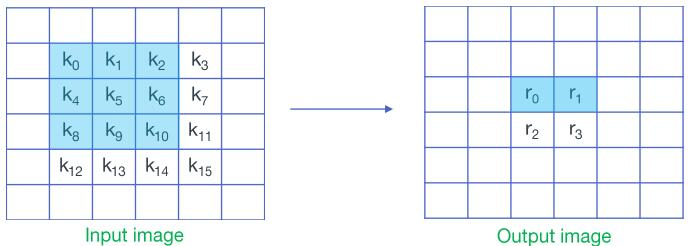$W = \left[\begin{array}{lll}{w_{0}} & {w_{1}} & {w_{2}} \\ {w_{3}} & {w_{4}} & {w_{5}} \\ {w_{6}} & {w_{7}} & {w_{8}}\end{array}\right]$
对直接卷积来说，该过程一共需要36次乘法和32次加法。
参考Even Faster CNNs: Exploring the New Class of Winograd Algorithms，将输入按滑窗分块后展开成向量并堆叠成矩阵，将权重展开成向量——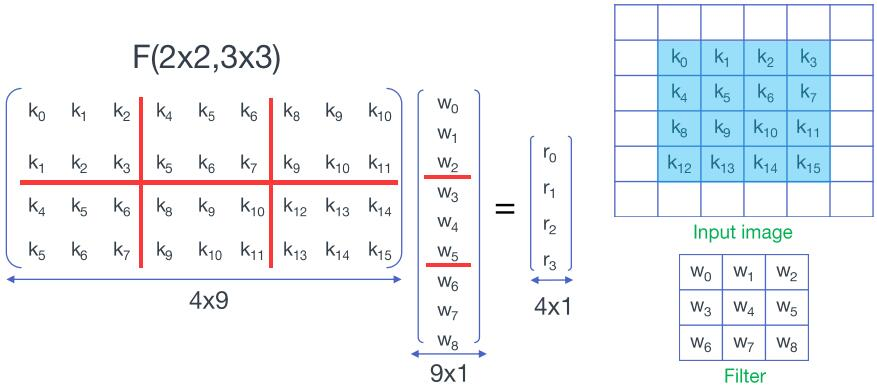对矩阵和向量进行分块——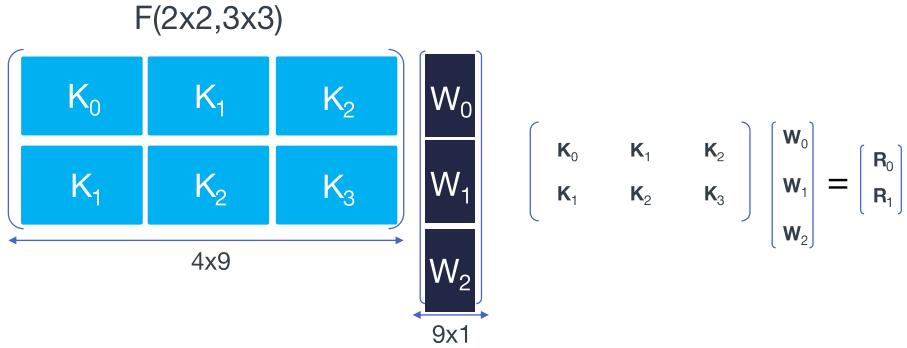堆叠实现
\begin{aligned} \left[\begin{array}{lll}{K_0} & {K_1} & {K_2} \\ {K_1} & {K_2} & {K_3} \end{array}\right] \left[\begin{array}{l}{W_0} \\ {W_1} \\ {W_2} \end{array}\right] &= \left[\begin{array}{l}{R_0} \\ {R_1} \end{array}\right] = \left[\begin{array}{l}{K_0W_0+K_1W_1+K_2W_2} \\ {K_1W_0+K_2W_1+K_3W_2} \end{array}\right] \\ \\ &= \left[\begin{array}{l}{F_{(2,3)}(D_0,W_0)+F_{(2,3)}(D_1,W_1)+F_{(2,3)}(D_2,W_2)} \\ {F_{(2,3)}(D_1,W_0)+F_{(2,3)}(D_2,W_1)+F_{(2,3)}(D_3,W_2)} \end{array}\right] \end{aligned}
其中，$D_i$是$K_i$对应的输入序列，也即卷积输入的第$i$行
$D = \left[\begin{array}{llll} {k_0} & {k_4} & {k_8} & {k_{12}} \\ {k_1} & {k_5} & {k_9} & {k_{13}} \\ {k_2} & {k_6} & {k_{10}} & {k_{14}} \\ {k_3} & {k_7} & {k_{11}} & {k_{15}} \end{array}\right] = \left[\begin{array}{l} D_0 & D_1 & D_2 & D_3 \end{array}\right]$
也就是说，$F(2\times2, 3\times3)$在这里分成了6次$F(2,3)$以及4次额外的加法，总计24次乘法和44次加法（注意：虽然这里做了6次$F(2,3)$但是输入序列的变换只需要做4次，所以加法次数是44次而非52次），相比于直接卷积的36次乘法和32次加法，乘法次数跟一维的$F(2,3)$一样，也下降为原来的$\frac{2}{3}$，同理也需要付出3倍于$F(2,3)$额外的空间代价（三个$W$）：
嵌套实现
\begin{aligned} \left[\begin{array}{lll}{K_0} & {K_1} & {K_2} \\ {K_1} & {K_2} & {K_3} \end{array}\right] &= \left[ \begin{array}{c}{R_0} \\ {R_1}\end{array}\right] = \left[ \begin{array}{c}{K_0 W_0 + K_1 W_1 + K_2 W_2} \\ {K_1 W_0 + K_2 W_1 + K_3 W_2} \end{array} \right] \\ \\ &= \left[\begin{array}{l}{F_{(2,3)}(D_0,W_0)+F_{(2,3)}(D_1,W_1)+F_{(2,3)}(D_2,W_2)} \\ {F_{(2,3)}(D_1,W_0)+F_{(2,3)}(D_2,W_1)+F_{(2,3)}(D_3,W_2)} \end{array}\right] \\ &= \left[ \begin{array}{c} {A^{T}\left[(G W_0) \odot\left(B^{T} D_0 \right)\right] + A^{T}\left[(G W_1) \odot\left(B^{T} D_1 \right)\right] + A^{T}\left[(G W_2) \odot\left(B^{T} D_2 \right)\right]} \\ {A^{T}\left[(G W_0) \odot\left(B^{T} D_1 \right)\right] + A^{T}\left[(G W_1) \odot\left(B^{T} D_2 \right)\right] + A^{T}\left[(G W_2) \odot\left(B^{T} D_3 \right)\right]} \end{array} \right] \\ \\ &=A^{T}\left[\left[G [W_0 \ W_1 \ W_2 ] G^{T}\right] \odot\left[B^{T} [D_0 \ D_1 \ D_2 \ D_3] B\right]\right]A \\ \\ &=A^{T}\left[\left[G w G^{T}\right] \odot\left[B^{T} d B\right]\right] A \\ \\ &\textit{(...w => g...)} \\ \\ &=A^{T}\left[\left[G g G^{T}\right] \odot\left[B^{T} d B\right]\right] A \end{aligned}
也即，
$F(2\times2, 3\times3) = A^{T} \left[ U \odot V \right] A$
其中，
$U = G g G^{T}$
$V = B^{T} d B$
与$F(2,3)$同理，可以推导出，$F(2\times2, 3\times3)$需要16次乘法和14次加法（$V=B^{T} d B$过程8次加法、$M=U \odot V$过程16次乘法、$Y=A^TMA$过程6次加法），相比于直接卷积的36次乘法和32次加法，乘法次数下降为原来的$\frac{16}{36}$。计算量的减少比堆叠实现要明显，但也需要更多的额外空间代价：直接计算只需要存储9个参数的$g$，$F(2\times2,3\times3)$则需要存储16个参数的$GgG^T$（推广到一般情况，分别为$r^2$和$(r+m-1)^2$）
$G$、$B^T$、$A^T$
Winograd算法需要推导出相应的变换矩阵$G$、$B^T$和$A^T$，但具体的推导过程似乎有些复杂，我现在还没弄懂。所幸 wincnn | github提供了一个解算$G$、$B^T$、$A^T$的工具，除了前述的$F(2,3)$，常用的还有$F(4,3)$和$F(6,3)$，它们对应的变换矩阵如下：

$F(4,3)$
$B^{T}=\left[\begin{array}{rrrrrr} {4} & {0} & {-5} & {0} & {1} & {0} \\ {0} & {-4} & {-4} & {1} & {1} & {0} \\ {0} & {4} & {-4} & {-1} & {1} & {0} \\ {0} & {-2} & {-1} & {2} & {1} & {0} \\ {0} & {2} & {-1} & {-2} & {1} & {0} \\ {0} & {4} & {0} & {-5} & {0} & {1} \end{array}\right], G=\left[\begin{array}{rrr} {1/4} & {0} & {0} \\ {-1/6} & {-1/6} & {-1/6} \\ {-1/6} & {1/6} & {-1/6} \\ {1/24} & {1/12} & {1/6} \\ {1/24} & {-1/12} & {1/6} \\ {0} & {0} & {1} \end{array}\right], \\ A^{T}=\left[\begin{array}{rrrrrr} {1} & {1} & {1} & {1} & {1} & {0} \\ {0} & {1} & {-1} & {2} & {-2} & {0} \\ {0} & {1} & {1} & {4} & {4} & {0} \\ {0} & {1} & {-1} & {8} & {-8} & {1} \end{array}\right]$
$g=\left[\begin{array}{lll}{g_{0}} & {g_{1}} & {g_{2}}\end{array}\right]^{T}, d=\left[\begin{array}{llllll}{d_{0}} & {d_{1}} & {d_{2}} & {d_{3}} & {d_4} & {d_5}\end{array}\right]^{T}$
$F(6,3)$
$B^{T}=\left[\begin{array}{rrrrrrrr} {1} & {0} & {-21/4} & {0} & {21/4} & {0} & {-1} & {0} \\ {0} & {1} & {1} & {-17/4} & {-17/4} & {1} & {1} & {0} \\ {0} & {-1} & {1} & {17/4} & {-17/4} & {-1} & {1} & {0} \\ {0} & {1/2} & {1/4} & {-5/2} & {-5/4} & {2} & {1} & {0} \\ {0} & {-1/2} & {1/4} & {5/2} & {-5/4} & {-2} & {1} & {0} \\ {0} & {2} & {4} & {-5/2} & {-5} & {1/2} & {1} & {0} \\ {0} & {-2} & {4} & {5/2} & {-5} & {-1/2} & {1} & {0} \\ {0} & {-1} & {0} & {21/4} & {0} & {-21/4} & {0} & {1} \end{array}\right], \\ G=\left[\begin{array}{rrr} {1} & {0} & {0} \\ {-2/9} & {-2/9} & {-2/9} \\ {-2/9} & {2/9} & {-2/9} \\ {1/90} & {1/45} & {2/45} \\ {1/90} & {-1/45} & {2/45} \\ {32/45} & {16/45} & {8/45} \\ {32/45} & {-16/45} & {8/45} \\ {0} & {0} & {1} \end{array}\right], \\ A^{T}=\left[\begin{array}{rrrrrrrr} {1} & {1} & {1} & {1} & {1} & {1} & {1} & {0} \\ {0} & {1} & {-1} & {2} & {-2} & {1/2} & {-1/2} & {0} \\ {0} & {1} & {1} & {4} & {4} & {1/4} & {1/4} & {0} \\ {0} & {1} & {-1} & {8} & {-8} & {1/8} & {-1/8} & {0} \\ {0} & {1} & {1} & {16} & {16} & {1/16} & {1/16} & {0} \\ {0} & {1} & {-1} & {32} & {-32} & {1/32} & {-1/32} & {1} \\ \end{array}\right]$
$g=\left[\begin{array}{lll}{g_{0}} & {g_{1}} & {g_{2}}\end{array}\right]^{T}, d=\left[\begin{array}{llllll}{d_{0}} & {d_{1}} & {d_{2}} & {d_{3}} & {d_4} & {d_5} & {d_6} & {d_7}\end{array}\right]^{T}$

注意：与$F(2,3)$不同，由于$F(4,3)$和$F(6,3)$的$A^T$、$B^T$出现了非$[0,1,-1]$的元素，所以除了点乘过程，还会引入额外的乘法运算。
比较
接下来我们对$F(2,3)$、$F(4,3)$、$F(6,3)$、$F(2\times2,3\times3)$、$F(4\times4,3\times3)$、$F(6\times6,3\times3)$的效果做一简单比较。

原始乘+加
Win乘+加
乘法加速比
乘法加速比（含变换）
原始内存
Win内存
内存增长

$F(2,3)$
6+4
4(4)+8
1.50
1.50
3
4
1.33

$F(4,3)$
12+8
12(6)+30
2.00
1.00
3
6
2.00

$F(6,3)$
18+12
28(8)+66
2.25
0.64
3
8
2.67

堆$F(2\times2,3\times3)$
36+32
24(24)+44
1.50
1.50
9
12
1.33

堆$F(4\times4,3\times3)$
144+128
126(72)+240
2.00
1.14
9
18
2.00

堆$F(6\times6,3\times3)$
324+288
404(144)+864
2.25
0.80
9
24
2.67

嵌$F(2\times2,3\times3)$
36+32
16(16)+14
2.25
2.25
9
16
1.78

嵌$F(4\times4,3\times3)$
144+128
48(36)+58
4.00
3.00
9
36
4.00

嵌$F(6\times6,3\times3)$
324+288
104(64)+134
5.06
3.12
9
64
7.11

以上数据是我自己手推的，可能有不正确的地方，欢迎指正~

Win乘+加列括号内表示不考虑变换产生的乘法运算（即仅考虑点乘的乘法）
注意，在$V = B^{T} d B$和$Y = A^T M A$的计算过程中，$A^T$或$B^T$矩阵内元素绝对值相同的乘法运算其实是可以合并的
从理论的乘法加速比来看（只考虑点乘的乘法），Winograd都有相当理想的加速效果，嵌套实现的$F(6\times6,3\times3)$甚至有5.06倍的加速
但考虑到$V = B^{T} d B$和$Y = A^T M A$的计算过程中也有大量的乘法，实际乘法加速效果并没有那么高，嵌套实现的$F(6\times6,3\times3)$其实也只有3.12倍的加速效果；$F(6,3)$和堆叠实现的$F(6\times6,3\times3)$反而出现明显的减速；
$F(2,3)$和$F(2\times2, 3\times3)$非常有意思，其$A^T$矩阵和$B^T$矩阵的元素均为$[0,1,-1]$——也就是说在变换过程中不会产生额外的乘法开销
从额外的内存开销上来看（仅考虑参数占用的内存），二维大于一维、嵌套实现大于堆叠实现
尽管$V = B^{T} d B$和$Y = A^T M A$的计算过程中也有大量的乘法，但观察可以发现$F(4,3)$和$F(6,3)$的$A^T$矩阵和$B^T$中有相当多的元素恰好是$2^n$，也就是说，用Winograd计算量化的卷积应该会有神奇的加成（对浮点运算来说，能否直接通过对指数部分做加法实现？？）


展开全文深度学习
• ## Caffe的卷积原理

万次阅读 多人点赞 2016-03-03 10:11:34
Caffe的卷积原理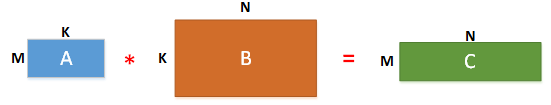Caffe中的卷积计算是将卷积核矩阵和输入图像矩阵变换为两个大的矩阵A与B，然后A与B进行矩阵相乘得到结果C（利用GPU进行矩阵相乘的高效性），三个矩阵的说明如下：
（1）在矩阵A中
M为卷积核个数，K=k*k，等于卷积核大小，即第一个矩阵每行为一个卷积核向量（是将二维的卷积核转化为一维），总共有M行，表示有M个卷积核。
（2）在矩阵B中
image_h：输入图像的高度
image_w：输入图像的宽度
kernel_h：卷积核的高度
kernel_w：卷积核的宽度
stride_h：高度方向的滑动步长；
stride_w：宽度方向的滑动步长。
因此，N为输出图像大小的长宽乘积，也是卷积核在输入图像上滑动可截取的最大特征数。
K=k*k，表示利用卷积核大小的框在输入图像上滑动所截取的数据大小，与卷积核大小一样大。
（3）在矩阵C中
矩阵C为矩阵A和矩阵B相乘的结果，得到一个M*N的矩阵，其中每行表示一个输出图像即feature map，共有M个输出图像（输出图像数目等于卷积核数目）

（在Caffe中是使用src/caffe/util/im2col.cu中的im2col和col2im来完成矩阵的变形和还原操作）

举个例子（方便理解）：
假设有两个卷积核为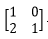与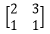，因此M=2，kernel_h=2，kernel_w=2，K=
kernel_h * kernel_w=4
输入图像矩阵为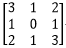，因此image_h=3，image_w=3，令边界扩展为0即pad_h=0，pad_w=0，滑动步长为1，即stride_h=1，stride_w=1
故N=[(3+2*0-2)/1+1]*[ (3+2*0-2)/1+1]=2*2=4

A矩阵（M*K）为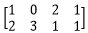，B矩阵（K*N）为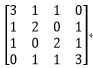C=A*B=*=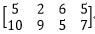C中的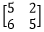与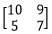分别为两个输出特征图像即featuremap。

在Caffe源码中，src/caffe/util/math_functions.cu（如果使用CPU则是src/util/math_functions.cpp）中的caffe_gpu_gemm()函数，其中有两个矩阵A（M*K）
与矩阵    B（K*N），大家可以通过输出M、K、N的值即相应的矩阵内容来验证上述的原理，代码中的C矩阵与上述的C矩阵不一样，代码中的C矩阵存储的是偏置bias，
是A  与B相乘后得到M*N大小的矩阵，然后再跟这个存储偏置的矩阵C相加完成卷积过程。如果是跑Mnist训练网络的话，可以看到第一个卷积层卷积过程中，
M=20，K=25，N=24*24=576。
（caffe中涉及卷积具体过程的文件主要有：src/caffe/layers/conv_layer.cu、src/caffe/layers/base_conv_layer.cpp、                src/caffe/util/math_functions.cu、src/caffe/util/im2col.cu）
另外大家也可以参考知乎上贾扬清大神的回答，帮助理解http://www.zhihu.com/question/28385679

注：如果本人理解有误，欢迎大家指出。

展开全文Caffe
• 数字图像卷积原理，matlab的conv2函数，不使用con2函数实现卷积 图像卷积原理 卷积，Convolution。卷积是两个变量在某范围内相乘后求和的结果。如果卷积的变量是序列x(n)和h(n)，则卷积的结果为： 本文只讨论卷积...
• 基于盲去卷积原理的图像复原程序代码， 露西-理查德森算法属于图像复原中的非线性算法，与维纳滤波这种较为直接的算法不同，该算法使用非线性迭代技术，在计算量、性能方面都有了一定提升。
• 文章目录反卷积原理首先来看卷积操作然后来看反卷积操作代码中的实现首先来看卷积的代码然后再来看反卷积结合代码看反卷积过程 反卷积原理 转载自该博文 反卷积（转置卷积、空洞卷积（微步卷积））近几年用得较多，...深度学习
• ## 反卷积原理

千次阅读 2018-05-13 15:17:20
这里千万不要当成反卷积操作可以复原卷积操作的输入值，反卷积并没有那个功能，它仅仅是将卷积变换过程中的步骤反向变换一次而已，通过将卷积核转置，与卷积后的结果再做一遍卷积，所以它还有个名字叫转置卷积。...
• keras 卷积原理分析 原文链接：https://blog.csdn.net/weixin_43654661/article/details/86070581 假设我们有这样一个2D卷积模型 modle = Sequential() model.add(Conv2D(32, (3, 3), activation='relu', input_...keras
• ## CNN卷积原理

千次阅读 2018-11-26 15:12:08
https://blog.csdn.net/qq_31456593/article/details/76083091 https://blog.csdn.net/qq_31456593/article/details/76083091 ... 一、卷积神经网络 1、简介 卷积神经网络最主要的特点就是局部...
• 转载自： &amp;...0、标准卷积 ...那么一般的操作就是用32个3×3的卷积核来分别同输入数据卷积，这样每个卷积核需要3×3×16个参数，得到的输出是只有一个通道的数据。之所以会得...
• ## 图像卷积原理及运算方法

万次阅读 多人点赞 2018-06-07 15:39:46
原理 卷积，有时也叫算子。用一个模板去和另一个图片对比，进行卷积运算。目的是使目标与目标之间的差距变得更大。卷积在数字图像处理中最常见的应用为锐化和边缘提取。 如边缘提取，假如目标像素点和它周边的值...
• ## Caffe卷积原理

千次阅读 2016-08-19 16:39:09
Caffe中的卷积计算是将卷积核矩阵和输入图像矩阵变换为两个大的矩阵A与B，然后A与B进行矩阵相乘得到结果C（利用GPU进行矩阵相乘的高效性），三个矩阵的说明如下： （1）在矩阵A中  M为卷积核个数，K=k*k，...
• 在深度学习的过程中，很多神经网络都会用到各种卷积核来进行操作，那么我们就简单讲一下卷积原理和实现过程。 那么卷积在神经网络中的作用是什么呢？一开始的传统神经网络是没有卷积层的，都是隐藏层加生全连接层...深度学习
• 二十、卷积原理与操作 神经网络 （1）准备数据； （2）前向传播，算出输出（分类结果/回归结果），得到损失函数（衡量对当前数据拟合的好坏）； （3）反向传播计算梯度值，看权重值分别对loss产生多大影响，看怎么改...
• 0、标准卷积 默认你已经对卷积有一定的了解，此处不对标准卷积细讲。 举个例子，假设有一个3×3大小的卷积层，其输入通道为16、输出通道为32。 那么一般的操作就是用32个3×3的卷积核来分别同输入数据卷积，这样每...
• 一：什么是卷积离散卷积的数学公式可以表示为如下形式：f(x) = - 其中C(k)代表卷积操作数，g(i)代表样本数据, f(x)代表输出结果。举例如下：假设g(i)是一个一维的函数，而且代表的样本数为G = [1,2,3,4,5,6,7,8,9]...
• 假设我们有这样一个2D卷积模型 modle = Sequential() model.add(Conv2D(32, (3, 3), activation='relu', input_shape=(150, 150, 3))) model.add(Maxpooling2D((2, 2))) model.add(Conv2D(64, (3, 3), activation='...
•  卷积卷积神经网络的重要组成部分，消耗整个网络中大量计算资源，理解卷积计算过程，对优化网络结构和简化网络模型非常重要。  正常卷积的实现如下图所示：  非常重要的是：卷积核其实和特征图一样，是一...
• 以上我们已经知道了卷积是如何操作的，im2col的作用就是优化卷积运算，如何优化呢，我们先学习一下这个函数的原理。 我们假设卷积核的尺寸为2*2，输入图像尺寸为3*3.im2col做的事情就是对于卷积核每一次要处理的小窗...目标检测
• 卷积（Deconvolution）的概念第一次出现是Zeiler在2010年发表的论文Deconvolutional networks中，但是并没有指定反卷积这个名字，反卷积这个术语正式的使用是在其之后的工作中(Adaptive deconvolutional networks ......